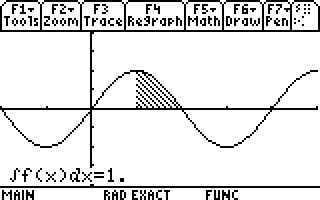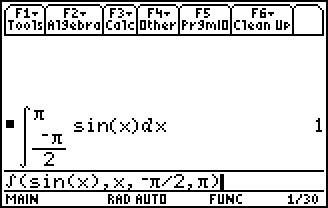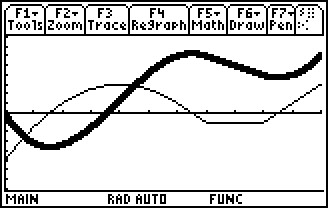# Activities

••• ##### Subject Area

• Math: Calculus: Applications of Integral, Antiderivatives and Definite Integrals
• Math: Calculus: Antiderivatives and Slope Fields

• ##### Author9-12

20 Minutes

• ##### Device
• TI-89 / TI-89 Titanium
• TI-92 Plus / Voyage™ 200
• ##### Report an Issue

Area Accumulation#### Activity Overview

Students explore the integral of a periodic function both numerically and graphically in order to gain an understanding of area accumulation.

#### Key Steps

•Students will numerically and graphically explore the integral using area accumulation.

•Students will use the handheld to explore what happens to the integral of sin(x)dx for a fixed value of a. Students will make observations about the integral for x < a, a = x, and a < x.

•In an extension to the activity, students will explore the kinematic relationship between the acceleration and the velocity of a moving object.